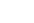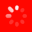The No.1 Website for Pro Audio
Gear Maniac

understanding dBV, dB, dBFS, dBSPL

Hey everyone,

I'm really struggling to grasp the concept of dB and all its different aplications.
I've read some "dB stickies", other threads and some websites (Sound On Sound) on this matter and, while some things have become more clear, others just won't get in my head!

From what I've been able to understand, decibel ( wether applied to power, voltage, sound pressure or headroom) is an "abstract" unit of measure.

if 0dBu is "set" to correspond to 0.775v, then -1dBu will correspond to whatever the logarithmic calculation tells me, right?

0dBV is commonly set to correspond to a voltage level of 1. as you go up or down the scale, logarithmic calculations tell you what voltage you're at.

Now, I'm looking at my mixer. One of the faders reads 0dB. If I move the fader down, what am I really doing? (besides lowering the output level)
Am I lowering voltage? current? or power?

I know this question reveals my lack of understanding regarding signal ouput (as in - is my mixers output power always the same? or does it vary depending on what's reading on my faders?)

as far as dBFS goes, I generally see people using this measure when talking about the meters in their daws. tipically, DAW peak meters read dBFS, right? do I need to relate these measures to my audio interfaces outputs? (will the output level be correctly read by the daw straight away?)

Sorry for the super messy post! It kinda reflects whats going on in my head! LOL

Thanks guyz.

GMX750Lives for gear

It may help if you come at this from a slightly different angle...

Think of the faders on your mixer as variable resistors - so moving them will adjust the flow (current = amperage) of the signal, and consequently also the voltage (Ohm's law V=IR).

dBFS is dB full scale, which is a measurement of dynamics - 0dBFS is the maximum threshold value in the scale before clipping occurs. 0 dBVU is a reference point that allows for a certain amount of headroom prior to clipping. If you have 12dB of headroom after 0 dBVU before clipping, then 0 dBVU is the same as -12 dBFS.

VU are volume units which have a non-relative measure, specifically 1.228VAC RMS across 600 ohms at 1kHz, which is less verbosely known as +4dBu (e.g. pro line level), but the notion of 0 dBVU has been loosened a bit over the years, and "0 on the meter" depends on calibration (10dBV consumer level is also common).

Hope this helps clear some things up!
Gear Maniac

Thanks for the reply. It's finally making some sense to me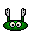How could I for example, make a correspondence between 0dB in my mixer and the actual dBV level that is being output? or dBSPL for that matter? the latter wouldn't make sense to me as a fixed value since SPL depends on the speaker, cabinet design and other external factors.

cheers

Just to expand a bit:
The Bel is a unit of measure named after alexander graham bel. it's too big for our purposes so we divide it by ten and get a deciBel.
It's a measure of RATIO, meaning that it is ALWAYS used to compare the measured value against something else. We append different suffixes depending on what we're using as the basis for comparison.

When used to measure POWER such as wattage or total acoustic power, a change of +3dB represents a doubling. The difference in power between a 100W amp and a 200W amp is 3dB. Also between a 25W light bulb and a 50W light bulb. (Doubling the power of your entire sound system only gives you 3dB more power, for the same reason.)

When used to measure VOLTAGE (the majority of audio signals) or pressure (such as a sound wave in air), a change of +6dB represents a doubling. So Increasing circuit voltage from 1V to 2V is a 6dB change. The faders on your console are measuring signal voltage which is why they're commonly labeled in 6dB gradations instead of 5 or 10.

dBFS (Full Scale) is a measure used in digital contexts to represent the maximum signal level before clipping is produced. This might be 22 volts for one device and 26 volts for another, but 0dBFS would be an accurate measure for each. dB is always relative!

dBV is referenced to 1 Volt. So 0dBV is 1V, 6dBV is 2 volts, -6dBV is .5V. (The scale is not linear, but logarythmic. It doesn't go 1,2,3,4,5, but 1,2,4,8,16. This is in part because it more accurately represents how the human ear responds to sound, and also because it lets us compress large ranges into smaller numbers. 80dB is a range of about 10,000 : 1.)

dBu is similar but it is referenced to .775V. This originates from the days of old 600 Ohm telephone transmissions. It is becoming less common but still pretty pervasive. The difference between 0dBu and 0dBV is 2.21dB. ( 1 Volt is 0dBV or 2.21dBu)

dBSPL (sound pressure level) is referenced to the quietest sound humans can hear, or the threshold of human hearing.

A fader is a RATIO control device. The numbers next to the fader show the ratio output to input. 0dB is no CHANGE in level through the fader. -6dB is an output of HALF the incoming voltage. -inf is no output, so infinitely less than the input.
Lives for gear

The dB is a logarithmic unit of ratios. What does this mean exactly? First, consider what a linear scale is: imagine you're charting your little cousin's height as he gets older. Every year you measure his height and write it down. Ten years go by and you decide to graph this as his birthday present. To create the graph, you put 10 equally-spaced tick marks on the horizontal axis and number them 0 through 9, each tick representing his age. On the vertical axis you put 10 equally-spaced ticks representing his height up to now. Finally, you add a dot for each height/age measurement you took and draw lines connecting them.

You've just created a linear graph: both the horizontal and vertical scales are linear. This means that between any two tick marks there is the same amount of "stuff" -- for example, between any two tick marks on the horizontal scale there is always one year's worth of time.

Now imagine that you're in audio engineering school and your professor has asked you to graph the relationship of loudness to certain events: a mosquito flapping its wing, a typical conversation, standing next to a jack hammer, and standing next to a nuclear explosion (if such a thing were possible). You do some research to find the pressure magnitude of these events and start building your graph, with the event on the horizontal axis and the pressure magnitude on the vertical axis. The horizontal axis is easy: four tick marks, each representing one of the events. But for the vertical axis, you realize you have a problem: the magnitude of a mosquito flapping its wing is on the order of 0.0001 pascals, the jack hammer around 2 pascals, and the nuclear explosion at about 100000 pascals. That means you need to show a range of 10^-5 to 10^5. If you make each tick equal to the smallest magnitude (10^-5), you'd need 10^10 ticks. That's 10 trillion ticks! (If you could manage to average 10 ticks per second, it would take you a little under 32 years to finish drawing the vertical axis. That's a lot of paper.)

Obviously this won't do. You need something manageable, say 10 horizontal tick marks. But how do you break up such a huge range into 10 chunks? If you try to make it a linear scale -- where each chunk has the same amount of "stuff" in it -- you run into another kind of problem: If each tick is worth 100000/10 = 10000 pascals, then the very first tick contains all the events except the nuclear explosion. Worst still, the first tick has so much stuff in it that you won't have a visual indication of the difference in loudness between a mosquito flapping its wing and a jack hammer. Considering the very audible difference between the two, a linear scale is therefore a poor choice.

This is one situation where logarithmic scales shine. The range of stuff we want to graph is between 10^-5 and 10^5; as said before, this is 10^10 worth of stuff: you need 10^5 stuff to get from 10^-5 to 10^0, and then another 10^5 to get from 10^0 to 10^5. Iinstead of dividing this huge range into ten even chunks, why not use the ten exponents as the tick marks? This would give us, starting from the botton: -5, -4, -3, -2, -1, 0, 1, 2 ,3, 4, 5 (which is eleven ticks, but that's ok). The mosquito wing (0.00001 = 10^-5) would correspond to the -5 tick, the conversation (0.001 = 10^-3) with the -3 tick, the jack hammer (2 = a bit more than 10^0) with the 0 tick, and the nuclear explosion (100000 = 10^5) with the 5 tick. In this scale we can visually see that the jack hammer is halfway between the mosquito wing and the nuclear explosion, which makes pretty good sense.

So what exactly did we do there? If you notice, the stuff between ticks is not evenly distributed: the farther you go up (on the vertical scale; or to the right, on a horizontal scale) the more "stuff" there is between the ticks. In this case, the amount of stuff between the 0 tick and the 1 tick is ten units (10^0 = 1 and 10^1 = 10); the amount of stuff between 1 tick and 2 tick is almost a hundred units (10^1 = 10 and 10^2 = 100); the amount of stuff between the 2 tick and 3 tick is almost a thousand units (10^2 = 100 and 10^3 = 1000); and so on. The magnitude of each tick increases by a factor of 10; this nonlinearity allows us to represent huge numbers with small numbers. In this particular case we used factors of 10, which means our scale was base-10 logarithmic.

Quick and easy math lesson: a logarithm is an exponent. Next time you see the word logarithm or logarithmic, just think to yourself "exponent". For example, what is the base-10 logarithm of 100000? A logarithm is just an exponent, so what exponent of 10 gives you 100000? 5, because 10^5 = 100000. Therefore log(100000) = 5. Simple as that. We can change the base of the logarithm, but again, logarithms are all about the exponent. So what is log(8) in base-2? Well, what exponent of 2 gives us the number 8? The answer is 3, because 2*2*2 = 2^3 = 8. Now it should be a bit easier to understand what a logarithmic scale means: it's just a scale based on exponents. And because exponents are multiplicative, they allow us to map a huge ranges of numbers to a much smaller range. This is the essence of the dB.

The other important aspect of the dB is that it is a ratio; it tells us the magnitude of a measurement relative to something. So when you read that a preamp has 60 dB of gain, what they're telling you is that whatever level the input signal has, the output signal can have 60 dB more -- the 60 dB is relative to the input signal. We know that the dB is logarithmic (based on exponents), so what does 60 dB mean in linear terms?

By definition, a dB is a decibel, meaning one-tenth of a bel, which is an archaic unit that nobody uses. But the bel is the simplest representation of a logarithmic scale: it is the logarithm of the ratio of two quantities. For example, imagine I have a radio transmitter that has 1 watt of transmitting power and my friend has one with 100 watts of power. What is the difference in power between the two? The ratio of powers is 100:1, so his radio has 100 times the power (linear scale) of mine. In the logarithmic scale, we take the base-10 log of 100: what power of 10 gives us 100? The answer is 2: log(100) = 2. This tells us that his radio has 2 bels more power than mine. But the bel is a bit too big for comfortable practical use, so we defined the decibel. If one radio is 2 bels more powerful than the other, and there are 10 decibels per bel, then that radio is 20 dB stronger than the other.

What does this teach us? A couple of things: specifically, that 100 times the power equals 20 dB greater power; more generally, if P2 is the power produced by one device and P1 is the power produced by another device, then a dB is equal to 10 * log(P2/P1). But notice that I'm specifically using the word power, and when describing preamp gain, we're talking about magnitudes of voltage not power. So what's the distinction?

To understand this first we need a quick lesson in electronics. You probably have heard of Ohm's Law, which relates voltage, current, and resistance/impedance in electrical circuits. The fundamental expression for Ohm's Law is that the current through a resistor is equal to the quotient of the voltage across the resistor and its resistance value. Mathematically, I = V/R. Another electrical fact is that the power dissipated by a resistor is equal to the product of the voltage across the resistor and the current through the resistor, or, mathematically: P = V*I. Replacing the current term I with its Ohm's Law expression we get P = V*V/R, or equivalently, P = V^2/R. In English, this states that power is proportional to the square of the voltage.

Back to the linear understanding of 60 dB of preamp gain. Earlier I said that dB in power equals 10 * log(P2/P1). But now I just claimed that P is proportional to V^2. This means that dB in voltage must be equal to 10 * log(V2^2/V1^2) which we can rewrite as 10 * log[(V2/V1)^2]. A wonderful mathematical property of logarithms is that the exponentiation reduces to multiplication, e.g.: log(2^3) = 3 * log(2). This means that our definition of voltage dB can be changed from 10 * log[(V2/V1)^2] to 20 * log(V2/V1).

So if my preamp says it has 100 times (linear) gain, how many dB is that? 20 * log(100) = 40 dB. That means that whatever the input signal is, if I crank the volume on the preamp, it will be 40 dB = 100 times more voltage than the original signal. To go the other way, from dB to linear, we can use the antilog formula: V2 = V1 * 10^(dB/20). For example, if our preamp has 60 dB of gain and I input a 1 millivolt signal, the output will be 0.001 * 10^(60/20) = 1 volt. Normalizing V1 to 1 we see that 60 dB of gain is equal to 1000 times the amplitude.

Notice that we've been using the "naked" dB; when the comparison is between two arbitrary things, such as the relative input and output levels of a preamp, we use dB without any suffix. However, certain standard measurements exist that we can compare things to. For example, you know that +4 dBu is the professional nominal operating level for line voltage. The little u is telling us that the measurement is relative to a standard measurement, namely 0 dBu = 0.775 volts rms. This needs to be perfectly clear: if you see a "naked" dB, it is the ratio between two arbitrary things; if you see a dB with a suffix, such as dBu (voltage) or dBm (power), it is the ratio of something to the standard defined by the suffix. So if I tell you that my preamp has 60 dB of gain and then ask you to tell me how much the voltage is at the output, you will correctly tell me "not enough information". All you can say is that whatever comes out will have 1000 times the amplitude of whatever goes in. But if I tell you that I have +4 dBu at the output of my preamp and I ask you what the voltage is, you can calculate a specific number: recalling the antilog formula, V2 = V1 * 10^(dB/20) we know that V2 is the output voltage, V1 is the reference voltage (0 dBu = 0.775 V rms), and we want +4 dB: V2 = 0.775 * 10^(4/20) = 1.23 V rms.

So in recap, a "naked" dB has no absolute meaning (though it can be worked out if enough information is known), while a "suffixed" dB always has an absolute meaning because it is in reference to some standard. The reason we have so many suffixes -- dBu, dBm, dBV, dBSPL, dBFS, etc. -- is because we have so many different kinds of things we want to measure. The only catch is to make sure you're referencing apples to apples. And for all that, it's hopefully clear that the simple reason we use logarithmic scales is because it makes the numbers easier to work with. With practice "thinking logarithmically" will become second-nature and you'll realize that it is indeed worth the effort.

Best of luck!
Lives for gear

That's amazingly generous of you. Just copied it to a text document for referral.
Lives for gear

And mono cluster also copied to text thanks!
Lives for gear

Knowledge hath been droppeth'd... great explanations mono and bogo!
Gear Maniac

Even without reading both replies from bogosort and monocluster (btw thank you very very much for having the patience to write such comprehensive replies) I know I'm gonna dive deep into those words as soon as I get home from work!

thanks again for the walls of text : )

I'll post back shortly

GMX750Lives for gear

Holy cow, you guys - those were great reads, monocluster & bogosport!

Thanks for the morning coffee talk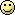Gear Maniac

I've finally managed to read the replies. Thank you for such clear and extensive information! I'm absolutely amazed.

I have some remaining doubts, hahah.
the 1 watt radio has 2 bel less power (watt =voltage x current) than the 100watt radio. you got to this value by using the base 10 log (100). why did you use the same base for calculating dB? wouldn't it be necessary to switch the base of the logarithm to represent a different scale?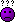you said 10 * log(p1/p2) is the dB difference in power between two sources.
Is this multiplication by 10 giving us the "deci" in bel?
Lives for gear

Quote:
I have some remaining doubts, hahah.
the 1 watt radio has 2 bel less power (watt =voltage x current) than the 100watt radio. you got to this value by using the base 10 log (100). why did you use the same base for calculating dB? wouldn't it be necessary to switch the base of the logarithm to represent a different scale?you said 10 * log(p1/p2) is the dB difference in power between two sources.
Is this multiplication by 10 giving us the "deci" in bel?
Great questions. For the first: All logarithms (in any base) share the same properties; changing the base merely scales the logarithm by some constant. The bigger the base, the more "squished" the logarithms are, but the logarithmic behavior is exactly the same. Thus we're free to choose the base of the logarithm using whatever is convenient for us. Mathematicians tend to use Euler's number 2.718... as the base because it simplifies the calculus, computer scientists invariably use base-2 -- computers are binary, after all -- and engineers prefer base-10 so we can use our fingers to count.So what's the difference between the bel and the decibel? Well they're really the same kind of thing, just different sizes: you need ten decibels to get one bel. Like teaspoons and tablespoons (in the US): you need 3 teaspoons to get one tablespoon. If a recipe called for two tablespoons of sugar and all you had was a teaspoon, you would just multiply how many "spoons" you need by three. Same with dBs: if log_10(100) = 2 bel but all you had are decibels, you just multiply by ten: 10 * log_10(100) = 20 dB.

Which should answer your second question: the multiplication by 10 "makes up" for the 1/10th of a bel value of a decibel.

Hope that makes it a little clearer. Let me know if not!
Gear Maniac

Quote:
Hope that makes it a little clearer. Let me know if not!
Yes!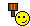man... thank you for the replies. you guys really helped me out here!If there's anything you guys need from over here at azores islands (a place to stay or some fine portuguese wine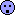) feel free to PM me at will! hehehe

Best of luck,
GMX750Lives for gear

Decibels are a way of expressing the ratio between two quantities.
Generally referenced against a fixed quantity. dbFS is decibels full scale, so the reference point is full scale, or the maximum possible level before distortion. Mathematically it is expressed as 20log(output level/input level) for voltages and 10log(Vout/Vin) for power.

The logarithmic scale is useful because it exploits a fundamental law of logarithms:
Log(10^2)=2xlog10. Or log(10^3)=3xlog10. So for a very large increase, for instance 10^10, which is 10,000,000,000. On the logarithm scale it is expressed as 10xlog10.
it's hard to explain without showing more examples of logarithmic behaviour but if your fader is at 0dB or unity gain the output voltage coming out of the that channel is the same as the voltage coming in to the fader from the previous gain stage on your console. Vout/Vin=1 and log1=0(dB). If your fader is at -60dB the output voltage is actually 1,000 times smaller than the input voltage: 20log(1/1,000)= -60dB.
it would be difficult to fit a million steps beside your fader so the log scale is convenient.
The 10 times multiplier at the front of the calculations for voltage is related to ohms law: V=IR to find the voltage, whereas for power the relationship is P=I^2R, again with power law of logarithms the square gets put out at the front of the equation and you get 10log(Vout/Vin) for power instead of 20log(Vout/Vin) for voltage.

hope some of this makes sense. It is a confusing business but it actually makes it much easier to show a very wide range of voltage on a small scale.
Here for the gear

I'm just going to leave this page open and read it every day for a while. Maybe one day I'll get it.
Lives for gear
This was posted recently…nice graph
Lives for gear

I wonder if using dB is useful because of the way we perceive sound. For instance, in the context of pitch we perceive the difference in pitch between the notes C2 and C3 to be equivalent to that of the notes C3 and C4, and yet numerically the latter is double the number of Hz, so the the dB scale is appropriate here.

I am guessing something similar happens with sound pressure level perception

I am also guessing that the various dB scale references were chosen to represent a sound pressure level that sits somewhere around the middle of the dynamic range of the human ear, or a voltage that produces such a sound pressure level when applied to certain "standard" equipment at the time.

Simplification and standards are generally a good thing, so I don't get what the bloody hell is the point in using so many different references. It makes things very confusing and in the end we are just trying to compare volts, watts or pascals. Imagine a grocery store where some items were labelled in Euros, some in Pounds, some in Dollars, some in Pesos, etc. I don't care which one you use but for goodness sake stick with one so we can compare!
Lives for gear

Excel calculator reference level

Created a little excel to calculate relation between dBFS, dBVU and dBu to see what happens when changing the reference level of the converter. It should show what happens to the headroom. I hope I understood it correctly.

Corrections and inputs are welcome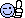Lives for gear

...oh, the file manager can't handle the new excel format. Here it is zipped.
Attached FilesCalculator-ReferenceLevel-dBFS_vs_dBVU_vs_dBu.zip (14.9 KB, 920 views)
Lives for gear

Thanks man, I was just about to do something like that. You just saved me some timeLives for gear

Quote:
Thanks man, I was just about to do something like that. You just saved me some timeYou're welcome. Tell me any bugs, features or if I did the calculation wrong.
Here for the gear

I knew I was in deep when I read the title of the post. Good information. I'm going to have to read this stuff over and over again so I get it. I like how the information is broken down so I can understand it.
Lives for gear

For all those who missed my Excel calculator above, here's a screenshot. Download the ZIP from post #19 (https://www.gearslutz.com/board/10101158-post19.html) and enter the reference level.

Thanks Macky, this is one crystal-clear graph, really helps to be able to visualize this stuff...I just need to learn it by heart now !
Lives for gear
So what does it mean in simple terms when you have a low 12 dB filter vs a low 24dB filter on a synth?
Lives for gear

Quote:
So what does it mean in simple terms when you have a low 12 dB filter vs a low 24dB filter on a synth?
If I understand your question correctly, you're talking about the filter slope, which is a function of the filter order. So let's say we have a low-pass filter with cutoff frequency of 1 kHz. A first-order filter will attenuate all frequencies above 1 kHz with a 6 dB per octave slope. An octave represents twice the frequency, so 2 kHz will be 6 dB down, 4 kHz will be 12 dB down, and so on.

Increasing the filter's order increases its slope (how fast it rolls off frequencies above the cutoff):

A second-order filter attenuates 12 dB per octave. So in our 1k LPF example, 2 kHz will be 12 dB down, 4 kHz will be 24 dB down, and so on.

A third-order filter has a slope of 24 dB per octave: 2 kHz will be 24 dB down, 4 kHz will be 48 dB down, and so on.
Lives for gear
Quote:
If I understand your question correctly, you're talking about the filter slope, which is a function of the filter order. So let's say we have a low-pass filter with cutoff frequency of 1 kHz. A first-order filter will attenuate all frequencies above 1 kHz with a 6 dB per octave slope. An octave represents twice the frequency, so 2 kHz will be 6 dB down, 4 kHz will be 12 dB down, and so on.

Increasing the filter's order increases its slope (how fast it rolls off frequencies above the cutoff):

A second-order filter attenuates 12 dB per octave. So in our 1k LPF example, 2 kHz will be 12 dB down, 4 kHz will be 24 dB down, and so on.

A third-order filter has a slope of 24 dB per octave: 2 kHz will be 24 dB down, 4 kHz will be 48 dB down, and so on.
So basically if I turn the knob from 2 to 1 khz it will go alot quieter alot quicker with the 24 db filter? Is that it? It has to do with the rate at which it's attenuating? That's what the main point of the two types of filters is? This has been one of the toughest things for me to learn with synths.
Lives for gear

Quote:
So basically if I turn the knob from 2 to 1 khz it will go alot quieter alot quicker with the 24 db filter? Is that it? It has to do with the rate at which it's attenuating? That's what the main point of the two types of filters is? This has been one of the toughest things for me to learn with synths.
Right, the rate of attenuation. So if it's a low-pass filter (lets the low frequencies through while blocking high frequencies), the 24 dB filter should sound darker than the 12 dB filter.
Lives for gear
Quote:
Right, the rate of attenuation. So if it's a low-pass filter (lets the low frequencies through while blocking high frequencies), the 24 dB filter should sound darker than the 12 dB filter.
Mmmhmmm...so if I want more violent type of fx combining with resonance then I should use 24, but if I want a more relaxed sound, less harsh I should use 12....

thanks....that makes sense, you know as much as you play around with knobs you still need to know the theory for some things to get it.
Gear Nut
Quote:
Simplification and standards are generally a good thing, so I don't get what the bloody hell is the point in using so many different references. It makes things very confusing and in the end we are just trying to compare volts, watts or pascals. Imagine a grocery store where some items were labelled in Euros, some in Pounds, some in Dollars, some in Pesos, etc. I don't care which one you use but for goodness sake stick with one so we can compare!
You actually said the key to it: "I don't care which one you use but for goodness sake stick with one so we can compare!". You need to have 2 values of the same unit, you can compare apples to apples, but not apples to oranges, that's why we use different types of dBs for different units: dBSPL for Pascals, dBv (and dBu) for volts, etc.
Topic:

## Welcome to the Gearslutz Pro Audio Community!

###### Registration benefits include:
• The ability to reply to and create new discussions
• Interact with VIP industry experts in our guest Q&As
• Get INSTANT ACCESS to the world's best private pro audio Classifieds for only USD \$20/year
• Remove this message!
You need an account to post a reply. Create a username and password below and an account will be created and your post entered.